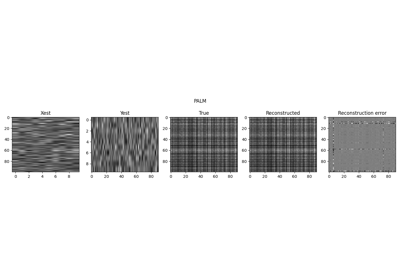# pyproximal.utils.bilinear.BilinearOperator#

class pyproximal.utils.bilinear.BilinearOperator[source]#

Common interface for bilinear operator of a function.

Bilinear operator template class. A user must subclass it and implement the following methods:

• gradx: a method evaluating the gradient over $$\mathbf{x}$$: $$\nabla_x H$$

• grady: a method evaluating the gradient over $$\mathbf{y}$$: $$\nabla_y H$$

• grad: a method returning the stacked gradient vector over $$\mathbf{x},\mathbf{y}$$: $$[\nabla_x H$$, [nabla_y H]

• lx: Lipschitz constant of $$\nabla_x H$$

• ly: Lipschitz constant of $$\nabla_y H$$

Two additional methods (updatex and updatey) are provided to update the $$\mathbf{x}$$ and $$\mathbf{x}$$ internal variables. It is user responsability to choose when to invoke such method (i.e., when to update the internal variables).

Notes

A bilinear operator is defined as a differentiable nonlinear function $$H(x,y)$$ that is linear in each of its components indipendently, i.e, $$\mathbf{H_x}(y)\mathbf{x}$$ and $$\mathbf{H_y}(y)\mathbf{x}$$.

Methods

 __init__() grad(y) gradx(x) grady(y) lx(x) ly(y) updatex(x) Update x variable (to be used to update the internal variable x) updatexy(xy) updatey(y) Update y variable (to be used to update the internal variable y)

## Examples using pyproximal.utils.bilinear.BilinearOperator`#Low-Rank completion via Matrix factorization

Low-Rank completion via Matrix factorization